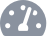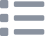# Change a Variable with a Potentiometer

## Change a variable with a potentiometer and ArduinoDifficulty
EasySteps
11

### Introduction

Potentiometers, also known as variable resistors, can be found in many Arduino projects.

In this guide, you will learn to use a potentiometer to turn values up and down and provide variable resistance. These values are read into the Arduino board as an analog input.

After completing this guide, you will be able to use potentiometers in your projects. Some examples of what you could do with a potentiometer are to control LEDs, MIDI or servos.

### Step 1   Insert our LED• Insert our LED so the Cathode (shorter leg) is on the left hand side.

### Step 2   Insert a 220 Ohm Resistor• Insert a 220 Ohm resistor so that one leg is in line with the positive leg of our LED.

### Step 3   Insert the Potentiometer• Insert the potentiometer so that the pins are aligned horizontally.

### Step 4   Connect LED Cathode to Ground• Connect the Cathode of our LED to a Ground pin on our Arduino.

### Step 5   Connect the 200 Ohm Resistor to Digital Pin 9• Connect the 200 Ohm Resistor to Digital Pin 9

### Step 6   Connect Potentiometer to 5V• Connect the first pin of the potentiometer to the Arduino's 5V pin.

### Step 7   Connect the Potentiometer to Ground• Connect the last pin on the potentiometer to the other Ground on the Arduino.

### Step 8   Connect the Potentiometer to Analog 0• Connect the middle signal pin of the Potentiometer to Analog pin 0

### Step 9   Upload this code

```int potPin = 0;    // Analog input pin that the potentiometer is attached to
int potValue = 0;  // value read from the pot
int led = 9;      // PWM pin that the LED is on.

void setup() {
Serial.begin(9600);
pinMode(led, OUTPUT);
}

void loop() {
analogWrite(led, potValue/4);  // PWM the LED with the pot value (divided by 4 to fit in a byte)
Serial.println(potValue);      // print the pot value back to the debugger pane
delay(10);                     // wait 10 milliseconds before the next loop
}```
• Copy this code to the Arduino IDE and upload it.
• Now when you turn the blue potentiometer the light should go from dull to bright!

### Step 10   Try this Code

```int sensorPin = A0;    // The potentiometer is connected to analog pin 0
int ledPin = 9;      // The LED is connected to digital pin 9
int sensorValue;	//We declare another integer variable to store the value of the potentiometer

void setup() // this function runs once when the sketch starts up
{
pinMode(ledPin, OUTPUT);
}

void loop() // this function runs repeatedly after setup() finishes
{